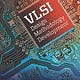# Reinforcement learning for logic synthesis

Logic synthesis is one of the most important steps in modern chip design, and consequently in EDA. Logic synthesis converts the behavioural level description into gate level description, which is one of the most important problems in EDA. Logic synthesis is the implementation of the specific logic functions by generating a combination of gates selected in a given cell library, and optimizes the design for different goals. Being a complicated process, it cannot be solved perfectly and so heuristic algorithms are widely used in this stage, which include lots of ML methods.

The emergence of new technologies and slowing down of Moore’s law is putting increasing pressure on the field of EDA. Logic synthesis requires extensive tuning of the synthesis optimization flow where the quality of results (QoR) is dependent on the sequence of optimizations used. Efficient design space exploration is challenging because of the exponential number of possible optimization permutations. There is a constant need to improve optimization algorithms, and it is necessary for these methods to be autonomous.

Here we examine two approaches for the same. Both approaches are given a Directed Acyclic Graph (DAG) as an input.
1) DRiLLS (Deep Reinforcement Learning based Logic Synthesis):

DRiLLS, which stands for Deep Reinforcement Learning-based Logic Synthesis, effectively maps the design space exploration problem to a game environment. Unlike most reinforcement learning environments where gamification drives the behavior of the environment, the task here involves combinatorial optimization the design of a given circuit. This makes it very challenging to not just define the state of the game (i.e. environment), but the long term incentives for an agent to explore the design space and not to fall into local minimums.

This method maps the complex search space to a “game” where an advantage actor critic (A2C) agent learns to maximize its reward (reduce area subject to a delay constraint) by iteratively choosing primitive transformations with the highest expected reward. There is a multi-objective reward function defined that takes into account the change in both the design area and the delay. In particular, the agent is rewarded for reducing the design area, while keeping the delay under a pre-defined constraint value.

2) GCN (Graph Convolution Networks):

This method suggests that the process of logic network optimization can be cast as a deterministic Markov Decision Process (MDP). Such a process can also be thought of as a single-player game of perfect information. The game consists of states and moves that transition from one state to the next one. The different game states correspond to logic networks. Moves in the game correspond to operations on the networks. In this approach, they use the majority inverter graphs (MIGs) as logic network data structures. MIGs correspond closely to a median (ternary majority) algebra, which allows to define a sound and complete move set. Such a move set allows it to reach any point in the design space. The policy function has been modelled as a deep neural network, based on Graph Convolution Networks (GCN). For every node in the MIG node features should be derived, based on a local neighbourhood, that capture information that is useful towards predicting which move is beneficial to apply.

Notice how both approaches take the form of a game. This is the beauty of reinforcement learning, where an agent is not told what action it should take, but instead receives a reward or penalty for actions. Autonomous learning of optimization is important and RL is an important step ahead.

## logic-synthesis-in-vlsi

This blog is about logic synthesis in vlsi, synthesis flow and machine learning functionality in logic synthesis## logic-synthesis-in-vlsi

This blog is about logic synthesis in vlsi, synthesis flow and machine learning functionality in logic synthesis